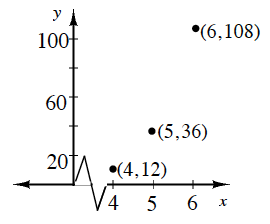### Home > CCA > Chapter Ch9 > Lesson 9.1.1 > Problem9-11

9-11.

Find an equation of the sequence represented by this graph.

How are the $y$-values changing?
Is the sequence arithmetic or geometric?

What is the common difference/multiplier?